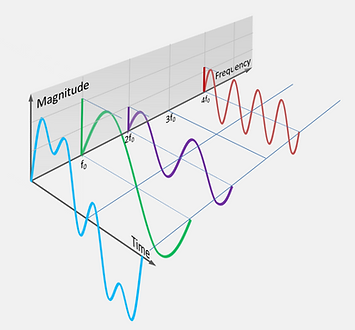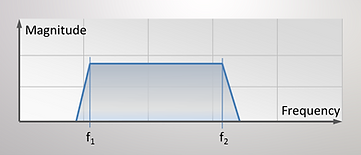What is Vibration?

# Basics of Vibration 3

## The Vibration Spectrum​

### ​​Frequency Analysis of Periodic Vibration

We have looked at sinusoidal vibration so far. Real vibration however normally consists of many different frequencies with different amplitudes occurring simultaneously. The vibration signal may then look like on the picture below.

Just by looking at a time signal we cannot immediately see how many components there are and at what frequencies they occur. It is not sinusoidal however it looks periodic!The French mathematician Joseph Fourier

has found out that every periodic signal can be split down in a number of sines and cosines at different magnitudes and frequencies the
"Fourier Series".

The fundamental frequency corresponds to the lowest frequency found in our signal. All other components appear at integral multiples of the fundamental and are called harmonics.Sample of a periodic vibration signal

The different components of the Fourier series can be calculated. Although very cumbersome you just need a large enough series of signal samples and apply the transformation formula.

The components are visualized by plotting the vibration magnitude against frequency instead of time.

This process is called frequency analysis. The picture opposite illustrates this. On the left side we find our periodic vibration signal and then the all the Fourier components as sinusoidal signals with their frequency and magnitude. The sum of all these sines gives the original signal again.Frequency analysis© by Wikipedia, the free encyclopedia

On the backside opf the 3-D presentation we see the graph that is obtained when we plot the magnitudes of all Fourier components against the frequency.

The graph showing the vibration level as a function of frequency is called a spectrogram or frequency spectrum.

We say also that a signal is represented in the frequency domain (versus time domain).Spectrogram of the vibration signal above

For the magnitude we can use any of the vibration terms be it acceleration, velocity or displacement.

### ​Frequency Analysis of Random Vibration

There is also vibration which is not periodic i.e. which has not a pattern that is repeated all along. For example if we drive along an unpaved road we will measure such a vibration. In the acoustic these signals are called noise.

For non periodic functions the Fourier transform is used instead of the Fourier series. The basic idea is thereby to interpret the non-periodic signal as a periodic one with the fundamental being infinite.

The picture shows such a typical random vibration signal. The peaks arrive in an unpredictable way.

In order to treat random vibration statistical laws are used but also the frequency spectrum found through the Fourier transform is a useful tool.Sample of a random vibration signalSpectrogram of a random vibration signal

While the individual sinus components
appear as separate lines in a spectrogram,

a random vibration appears as a surface.

It can extend across a wide range of

frequencies or it may consist of a narrow

band between the frequencies f1 and f2.

For the magnitude (the vertical dimension of

the surface) we cannot use the dimension of

the original signal in the time domain because we dont have distinct frequencies in the spectrum. Instead we use the power of the signal in a frequency range.

The power of the signal (which goes with the amplitude squared) is represented by the surface. It is for example expressed in g² . This means the magnitude becomes g² / Hz. We call it the power
spectral density. The "Hz" in this dimension is referring to the bandwidth we are looking at rather than
to the frequency on the x-axis.

The " g² " is  really a " g RM " but the RMS is normally omitted.

In order to reconstitute the original signal from the spectrum we need to apply an operation which is called Inverse Fourier Transform. This operation provides individual points (samples) of the original curve in the time domain.

### The Fast Fourier Transform FFT

The frequency analysis is a very important method in the diagnostic vibration measurements. When applied to a real machine vibration signal we normally find a number of predominant periodic frequency components which are directly related to the fundamental (cyclic) movements of various parts of the machine. With the frequency analysis we are therefore able to "look" into the machine and diagnose it down to individual parts.

In practical life in order to speed up the transformation time the fast Fourier transform (FFT) was invented. This is an algorithm which through clever data handling leads much quicker to the same result as the discrete Fourier transform. The FFT is used today throughout in the vibration analysis tools. We can reconstitute the original signal by applying the "inverse FFT".

Although the FFT is a clever tool it can also introduce errors in the analysis due to the start and stop of the sampling time and when the sampling time is not an integral multiple of the period of the fundamental. This may introduce a step in th etime function which is also FFT analysed and then leads to additional frequencies (artefacts) which were not in the original signal. To reduce the effect of this problem several (time-) windows are used such as "Flat-Top"; "Hamming"; "Blackmann -Harris"; "Hann"; etc. The artefacts may however still be present to some extent. This is why every frequency analysis although quickly done should be carefully interpreted.